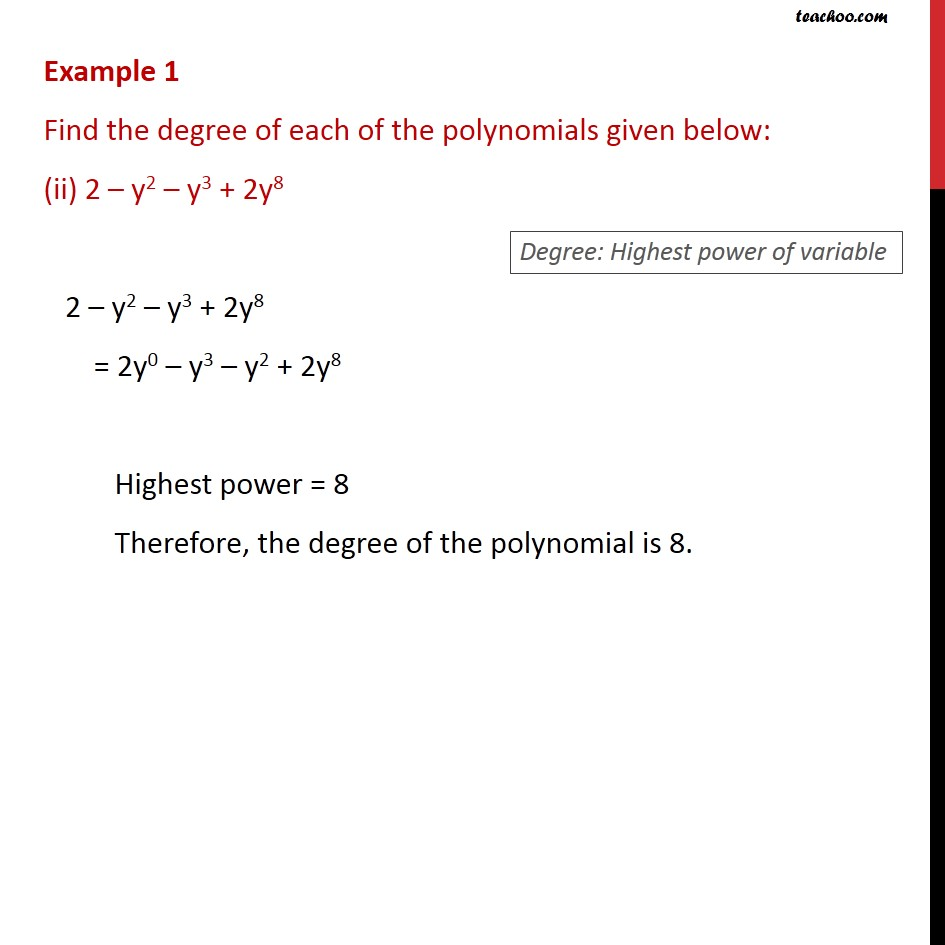1. Chapter 2 Class 9 Polynomials (Term 2)
2. Serial order wise
3. Examples

Transcript

Example 1 Find the degree of each of the polynomials given below: (ii) 2 y2 y3 + 2y8 2 y2 y3 + 2y8 = 2y0 y3 y2 + 2y8 Highest power = 8 Therefore, the degree of the polynomial is 8.